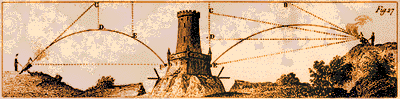### Language Challenge 2000The entire onboard software for the Apollo Lunar Landing Mission was about 10% the size of today's typical word processor -- a huge regress by any standard. For a measure of progress in scientific software you are invited to participate in a comparative test by solving a real world problem in the language of your choice. Find the optimal initial angle for a trajectory to reach a target at 2000 m to within 0.5 m. The equations of motion are given by, mx" + Dcos(alfa) = 0 my" + Dsin(alfa) + mg = 0 where D = .5*Cd*A*rho(y)*v2     alfa = atan(y'/x')     v = sqrt(x'2 + y'2) and rho() is true atmospheric density varying with altitude (use 1976 International Standard Atmosphere data).

 Parameters: m = 20 kg Cd = 0.3 A = 0.02 m2 g = 9.80665 m/s2 Initial values: x = 0 m y = 0 m v = 180 m/s alfa = 40 deg

 Rules: Solution must be general; trial and error methods based on apriori knowledge of the solution range do not qualify. Feel free to use external software resources as well as the "Trajectory" example on our "Application profiles" page as a convenient guideline. Criteria: a) Execution time b) Size of executable Submit: PC executable module (show final angle and distance), main program text file and indicate the integration and optimization algorithms used. e.g. Levenberg-Marquardt (netlib). All entries must be received by April 14, 2000. The best entry in each language will be posted on our site to serve as a barometer for those pondering what language to choose for their technical computing. It might also double as a place where flame war enthusiasts can calibrate their rhetoric against the realities of feasible solutions. The overall winner will receive: Compaq Visual Fortran V6.1 Professional Edition - Provided courtesy of Compaq SDX Modeling and Simulation Software V4.0 as recognition for demonstrated skills in crafting the fastest solution with the smallest footprint. Questions and submissions regarding the contest should be directed to info@sdynamix.com with Contest 2000 in the "Subject" line. Contest SummaryWe didn't think it was possible to silence the language warriors, however, the emptiness in the table suggests just that. What can be inferred from this deafening silence when the winning entry indicates that a quick top down design did the trick?. True, this was more than just a programming exercise, nevertheless, is the necessary know-how confined only to those who practice the language perceived by some as passé? This was an opportunity to show your prowess, not with rhetoric but with deeds -- code solving a real life problem -- yet you came up empty. There's an odd chance we've under estimated your technical savvy. You knew that if a certain language showed up you wouldn't have a chance, sort of like showing up at the Formula I race in a beat up bus? While we contemplate the reasons behind the blanks in the table, let the winning entry speak for itself.

### Results

 ada asm c c++ fortran pascal python vbasic other 1.29 2.28 2.52 2.83 1.07 1.68

### Winning Solution

Dr.Ing. Alexa Mihailovic, Zuerich, Switzerland

```

program trajectory
*---------------------------------------------------------*
*          find optimum alfa for target at 2km            *
*---------------------------------------------------------*
external fcn
common xhit
parameter (m = 1, n = 1)
real*8 alfa(n),fvc(m),tol
data tol/.25e-3/, alfa/40/

c.. least squares (Levenberg-Marquardt)
call lema (fcn,m,n,alfa,fvc,tol)
write(*,'(a,f9.3,a)') ' alfa =',alfa,' deg',' dist =',xhit,' m'
end

subroutine fcn (m,n,alfa,fvc)
external rate
common xhit
parameter (ns = 4)
real*8 alfa(n),fvc(m)
real x(ns)
equivalence (x(1),dist),(x(2),alti),(x(3),vx),(x(4),vy)
data d2r/1.745329e-2/, target/2000/, v/180/

c.. initial state
dist = 0
alti = 0
vx   = v*cos(alfa(1)*d2r)
vy   = v*sin(alfa(1)*d2r)

c.. integrate de (modified Euler-Cauchy)
do while (alti .ge. 0.)
call eca (rate,x,ns)
enddo

c.. estimate error
xhit   = dist - alti*vx/vy
fvc(1) = xhit - target
end

subroutine rate (x, dx)
real x(*),dx(*),
&     mass/20/, cd/.3/, area/.02/, g/9.80665/
rho(h) = (1.04884 - 2.365941e-5*h)**4.25588

c.. equations of motion
v     =  sqrt(x(3)**2 + x(4)**2)
drag  = .5*cd*area*rho(x(2))*v/mass

dx(1) =  x(3)
dx(2) =  x(4)
dx(3) = -drag*x(3)
dx(4) = -drag*x(4) - g
end

```

Q
Is the Standard Atmosphere already in "accepted formulas" or does one have to do a table look-up, interpolatory approximation, lsq or spline approximation in order to "guarantee" your targeting error?

A  Limiting the suggestion to using the Standard Atmosphere was deliberate as we did not want to prejudice design trade off considerations which you have effectively enumerated above. However, not finding the data wasn't meant to be the eliminating factor, so here is a relevant site:

Q  Based on your equations of motion, I would think that you have the constraint v = sqrt(x'^2 + y'^2) but you don't explicitly say it, leading the casual observer to think that the velocity along the trajectory is constant?

A  Indeed, one can never assume it's obvious to everyone. In fact, this "omission" would have lead to unwittingly missing a target entirely -- problem equations have been amended accordingly.

Q  Assuming there are two solutions (a high trajectory and a low one) do you care which one you get?

A  No we don't, but we suspect you might! It is also true that the initial angle is prescribed and if your optimization happens to stray into unwanted regions then adjusting algorithm parameters may well be worth a little extra scrutiny.

Q  It is unclear what exactly "generalized" is supposed to mean. Is it supposed to be completely generalized, generalized to problems of this type, or generalized to this problem's parameters.

A  The second characterization about covers it -- you have to build an optimization based solution that could be reused by simply substituting the eom and its performance measure. It was meant to exclude quick trial & error searches, root solvers or any other technique based on problem specific apriori knowledge (e.g. solution range, scalar dimension).